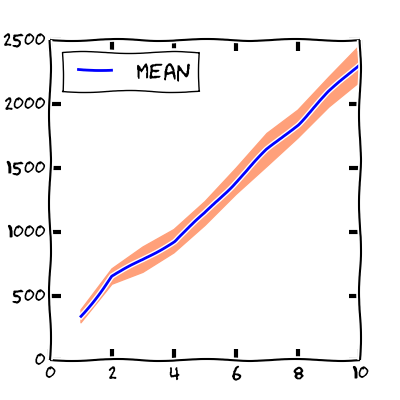One of the reasons for writing concurrent programs is to optimize the execution time. An essential part of the optimization is measuring.

Has concurrent program better performance? Is the multithreaded version faster than the single threaded? How does performance scale if we use more threads? Measuring the execution time can answer such questions.

In this article, we will look at some built-in techniques for measuring time and benchmarking.

# std::chrono

The standard way to measure time in C++ is to use the `<chrono>` standard library.

The library has several functions which returns current time. The most appropriate function for measuring the time intervals is the `std::chrono::steady_clock`. The reason is that the time of this clock can not decrease as the time moves forwards. The clock does not reset itself, therefore it is always monotonic.

Let’s say that we would like to measure the time needed to sum one million elements of a vector. We can measure it with the function `std::chrono::steady_clock::now()`, which returns current value of the clock.

The ```std::chrono::duration_cast< time_t >``` takes a duration and converts it to the duration of the type `time_t`. The duration time could be anything from nanoseconds to hours.

With these types and the two times `t1`, `t2`, we can calculate the duration in several units.

In my machine the printing

produces

# Simple benchmarking

Running the upper snippet of code several times will produce similar but a bit different results. This is completely normal. It is a consequence of operations of the inner parts of the computer.

In order to get more accurate results, we should perform measurement several times. Then, we should compute the average/mean of all measurements and the standard deviation. The standard deviation tells us how are the measurements spread around the mean value. If the standard deviation is small, all measurements are spread around the mean value. Otherwise, if the deviation is big, then the measurements are spread over a wide area around the mean.

There are people who recommend to throw away certain number of the initial measurements, because the initial ones might be less accurate then the following. Naively, we can imagine this effect as warming up the computer to its working temperature :-).

Let’s write a simple class, which will benchmark the execution time of a function call. The class declaration is:

The class has a template parameter `TimeT` which determines the measuring units.

The constructor accepts two arguments:

• `num_iterations` - the number of measurements,
• `throw_away` - the number of measurements, which will be thrown away.

The `Benchmark::benchmark` accepts a function `fun` and all of its arguments `args`. The member function measures the execution time of the input function ```(num_iterations + throw_away)``` times.

The `Benchmark::benchmark` returns the results of each execution of the function. There are two reasons for returning the results.

• If we are benchmarking concurrent function, we can check if the function returns correct results. If the results are different, we might have a data race.
• When we return results, the compiler can not optimize away the function call.

If the syntax of the `Benchmark::benchmark` declaration is not familiar to you, look at Variadic number of arguments and Return type - Part 1.

Additional public member functions are `mean()` and `standard_deviation()`. They are accesors to the average (mean) and standard deviation of all time measurements.

You can look at the entire source code of the class here.

# Simple example

Let’s use the class for benchmarking `std::accumulate`. We expect that the execution time increases linearly with respect to the number of elements.

The source code is available below.

The main function loops over a different number of elements. The body of the loop constructs the `std::vector` and benchmarks the `std::accumulate` on the vector. In each iteration, the loop prints the number of elements, the mean and the standard deviation of all measurements.

The output of the program is:

We can also visualize the output with a graph.The x-coordinate represents the number of elements of the vector (in millions) and the y-coordinate shows the microseconds.

The blue line marks the mean of all time measurements. The pink area around the mean indicates the region between `mean - standard_deviation` and `mean + standard_deviation`. We see that the mean grows linearly with respect to the number of elements. When increasing the number of elements also the standard deviation increases.

# Summary

We learned the basics of measuring the execution time.